## ICSE Chemistry Previous Year Question Paper 2018 Solved for Class 10

General Instruction:

• Answers to this Paper must be written on the paper provided separately.
• You will not be allowed to write during the first 15 minutes.
• This time is to be spent in reading the Question Paper.
• The time given at the head of this paper is the time allowedfor writing the answers.
• Section I is compulsory. Attempt any four questions from Section II.
• The intended marks for questions or parts of questions are given in brackets [ ].

Section -1 [40 Marks]
(Attempt ALL questions from this Section)

Question 1.
(a) Choose the correct answer from the options given below : 
(i) The salt solution which does not react with ammonium hydroxide is :
(A) Calcium Nitrate
(B) Zinc Nitrate
(D) Copper Nitrate
(A) Calcium Nitrate

(ii) The organic compound which undergoes substitution reaction is :
(A) C2H2
(B) C2H4
(C) C10H18
(D) C2H6
(D) C2H6

(iii) The electrolysis of acidified water is an example of:
(A) Reduction
(B) Oxidation
(C) Redox reaction
(D) Synthesis
(C) Redox reaction

(iv) The IUPAC name of dimethyl ether is:
(A) Ethoxy methane
(B) Methoxy methane
(C) Methoxy ethane
(D) Ethoxy ethane
(B) Methoxy methane

(v) The catalyst used in the Contact Process is :
(A) Copper
(B) Iron
(D) Manganese dioxide

(b) Give one word or a phrase for the following statements: 
(i) The energy released when an electron is added to a neutral gaseous isolated atom to form a negatively charged ion.
(ii) Process of formation of ions from molecules which are not in ionic state.
(iii) The tendency of an element to form chains of identical atoms.
(iv) The property by which certain hydrated salts, when left exposed to atmosphere, lose their water of crystallization and crumble into powder.
(v) The process by which sulphide ore is concentrated.
(i) Electron affinity
(ii) Ionisation
(iii) Catenation
(iv) Efflorescence
(v) Froth floatation process.

(c) Write a balanced chemical equation for each of the following : 
(i) Action of concentrated sulphuric acid on carbon.
(ii) Reaction of sodium hydroxide solution with iron (III) chloride solution.
(iii) Action of heat on aluminium hydroxide.
(iv) Reaction of zinc with potassium hydroxide solution.
(v) Action of dilute hydrochloric acid on magnesium sulphite.
(i) C + 2H2SO4 → 2H2O + 2SO2↑ + CO2
(ii) FeCl3 + 3NaOH → Fe(OH)3 + 3Nacl
(iii)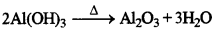(iv) Zn + 2KOH  → K2ZnO2 + H2O
(v) MgSO3 + 2HCl → MgCl2 + H2O + SO2

(d) (i) Give the IUPAC name for each of the following:(ii) Write the structural formula of the two isomers of butane.
(1) Methanal
(2) Propanol
(3) But-2-ene
(ii)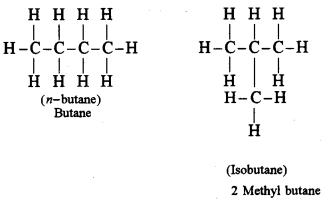(e) State one relevant observation for each of the following : 
(i) Lead nitrate solution is treated with sodium hydroxide solution drop wise till it is in excess.
(ii) At the anode, when molten lead bromide is electrolyzed using graphite electrodes.
(iii) Lead nitrate solution is mixed with dilute hydrochloric acid and heated.
(iv) Anhydrous calcium chloride is exposed to air for sometime.
(v) Barium chloride solution is slowly added to sodium sulphate solution.
(i)When drop by drop of sodium hydroxide is added, chalky white precipitates appear. These precipitates dissolve in excess of sodium hydroxide forming sodium plumbite.
(ii)At Anode
2Br– 2e → 2Br
Br+Br → Br2
At anode reddish vapours of bromine escape in air from lead bromide.
(iii)White precipitates of lead chloride are formed which are soluble in hot water and insoluble in cold water.
(iv) Anhydrous calcium chloride absorbs moisture becomes moist and loose its crystalline form showing its deliquescent nature.
(v) BaCl2+Na2SO4 → BaSO4+2NaCl

(f) Give a reason for each of the following: 
(i) Ionic compounds have a high melting point.
(ii) Inert gases do not form ions.
(iii) Ionisation potential increases across a period, from left to right.
(iv) Alkali metals are good reducing agents.
(v) Conductivity of dilute hydrochloric acid is greater than that of acetic acid.
(i) Ionic compounds have ions held strongly by electrostatic forces of attraction. These strong forces need more energy to be broken apart. Hence, they have high melting point.
(ii) Inert gases do not form ions because they have completely filled octet. They are extremely stable. Hence, they neither loose, nor gain electrons.
(iii) As we move from left to right along a period, the atomic size decreases due to the increase in nuclear charge thus more energy is required to remove the electron, hence ionisation potential increase.
(iv) Alkali metals have free electrons. They can easily loose electrons to form positive ions. The loss of electron is known as oxidation and the substance/element that lose electrons is said to be reducing agent.(v) Hydrochloric acid is a strong acid. It splits to give more hydrogen ions as compared to acetic acid. Hence conductivity of dilute hydrochloric acid is more than that of acetic acid.

(g) Name the gas that is produced in each of the following cases : 
(i) Sulphur is oxidized by concentrated nitric acid.
(ii) Action of dilute hydrochloric acid on sodium sulphide.
(iii) Action of cold and dilute nitric acid on copper.
(iv) At the anode during the electrolysis of acidified water.
(v) Reaction of ethanol and sodium.
(i) Nitrogen dioxide
(ii) hydrogen sulphide
(iii) nitrogen monoxide
(iv) oxygen
(v) hydrogen

(h) Fill up the blanks with the correct choice given in brackets. 
(i) Ionic or electrovalent compounds do not conduct electricity in their …………………. state. (fused/solid)
(ii) Electrolysis of aqueous sodium chloride solution will form …………………. at the cathode. (hydrogen gas/sodium metal)
(iii) Dry hydrogen chloride gas can be collected by …………………. displacement of air. (downward/upward)
(iv) The most common ore of iron is …………………. .(calomine / haematite)
(v) The salt prepared by the method of direct combination is ………………….. (iron (II) chloride / iron (III) chloride)
(i) fused
(ii) hydrogen gas
(iii) upward
(iv) haematite
(v) Iron (III) chloride

Section-II [40 Marks]
(Attempt any four questions from this Section)

Question 2.
(a) (i) What do you understand by a lone pair of electrons ? 
(ii) Draw the electron dot diagram of Hydronium ion. (H = 1,O = 8)
(i) The unshared pair of electron that does not normally take part in a chemical reaction is known as lone pair.(ii) Hydronium ion
Formation of proton:(b) In Period 3 of the Periodic Table, element B is placed to the left of element A. 
On the basis of this information, choose the correct word from the brackets to complete the following statements:
(i) The element B would have (lower/higher) metallic character than A.
(ii) The element A would probably have (lesser/higher) electron affinity than B.
(iii) The element A would have {greater/smaller) atomic size than B.
(i) Higher
(ii) higher
(iii) smaller

(c) Copy and complete the following table which refers to the conversion of ions to neutral particles.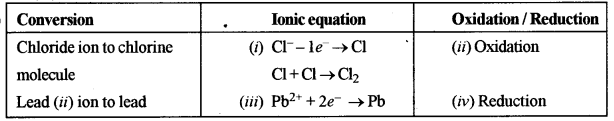Question 3.
(a) (i) Write the balanced chemical equation to prepare ammonia gas in the laboratory by using an alkali. 
(ii) State why concentrated sulphuric acid is not used for drying ammonia gas.
(iii) Why is ammonia gas not collected over water ?
(i)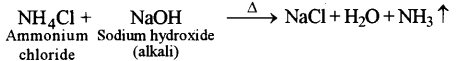(ii) Concentrated sulphuric acid is not used for drying ammonia gas because it reacts with ammonia.
2NH3 + H2SO4 → (NH4)2SO4
(iii) Ammonia is highly soluble gas one volume of water can dissolve 702 volumes of ammonia at 20°C and at 1 atmospheric pressure. Hence it is not collected over water.

(b) (i) Name the acid used for the preparation of hydrogen chloride gas in the laboratory. Why is this particular acid preferred to other acids ? 
(ii) Write the balanced chemical equation for the laboratory preparation of hydrogen chloride gas.
(b) (i) Sulphuric acid
[It is preferred to other acids because it is non-volatile acid]
(ii)(c) For the preparation of hydrochloric acid in the laboratory : 
(i) Why is direct absorption of hydrogen chloride gas in water not feasible ?
(ii) What arrangement is done to dissolve hydrogen chloride gas in water ?
(i) Direct absorption of hydrogen chloride gas in water is not feasible as it leads to back
suction.
(ii) “Inverted funnel arrangement” is done to dissolve hydrogen chloride gas in water.

(d) For the electro-refining of copper 
(i) What is the cathode made up of ?
(ii) Write the reaction that takes place at the anode.
(i) Cathode is made-up of thin sheets of pure copper Connected in parallel.
(ii) Cu – 2e → Cu2+
Copper anode undergoes oxidation forming Cu2+ ions which pass into the solution.

Question 4.
(a) The percentage composition of a gas is : 
Nitrogen 82.35%, Hydrogen 17.64%
Find the empirical formula of the gas. [N = 14, H = 1]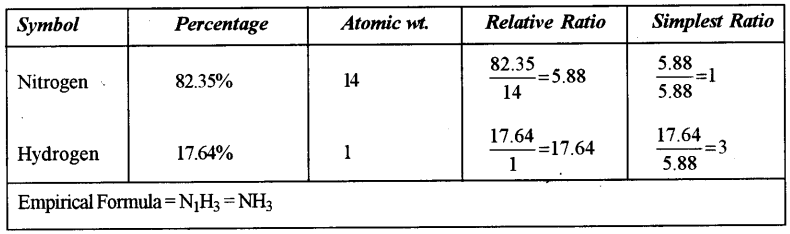(b) Aluminium carbide reacts with water according to the following equation: 
Al4C3 + 12H2O  → 4Al(OH)3 + 3CH4
(i) What mass of aluminum hydroxide is formed from 12 g of aluminum carbide ?
(ii) What volume of methane at s.t.p. is obtained from 12 g of aluminum carbide ?
[Relative molecular weight of Al4Cl4 = 144; Al(OH)3 = 78]
(i)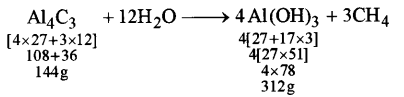144 g of Al4Cl3 produce 312 g of Al(OH)3
1 g of Al4C3 produce $$\frac{312}{144}$$ g of Al(OH)3
12g of Al4C3 produce $$\frac{312}{144}$$ × 12 = 26 g of Al(OH)3
(ii)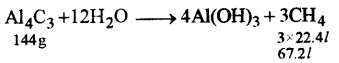144 g of Al4C3 will produce 67.2 / of methane
1 g of Al4C3 will produce $$\frac{67.2}{144}$$ l of methane
12 g of Al4C3 will produce $$\frac{67.2}{144} \times 12=5.6 g$$

(c) (i) If 150 cc of gas A contains X molecules, how many molecules of gas B will be present in 75 cc of B ? 
The gases A and B all under the same conditions of temperature and pressure.
(ii) Name the law on which the above problem is based.
(i) 150 cc of gas A contain X molecules
150 cc of gas B will also contain X molecules
75 cc of gas B will contain $$\frac{\mathbf{X}}{2}$$ molecules under the same conditions of temperature and pressure.

(d) Name the main component of the following alloys : 
(i) Brass
Brass → Copper, zinc and tin
Duralumin → Aluminium, copper, magnesium and manganese

Question 5.
(a) Complete the following table which relates to the homologous series of hydrocarbons.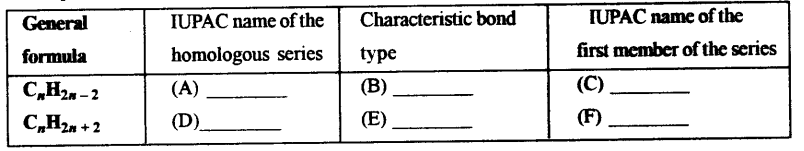(A) Alkynes
(B) triple bond
(C) ethyue
(D) Alkane
(E) single bond
(F) methane

(b) (i) Name the most common ore of the metal aluminum from which the metal is extracted. Write the chemical formula of the ore. 
(ii) Name the process by which impure ore of aluminum gets purified by using concentrated solution of an alkali.
(in) Write the equation for the formation of aluminum at the cathode during the electrolysis of alumina.
(i) Bauxite (Al2O3.2H2O)
(ii) Baeyer’s process
(iii)Question 6.
(a) A compound X (having vinegar like smell) when treated with ethanol in the presence of the acid Z, gives a compound Y which has a fruity smell. 
The reaction is: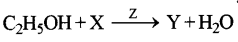(i) Identify Y and Z.
(ii) Write the structural formula of X.
(iii) Name the above reaction.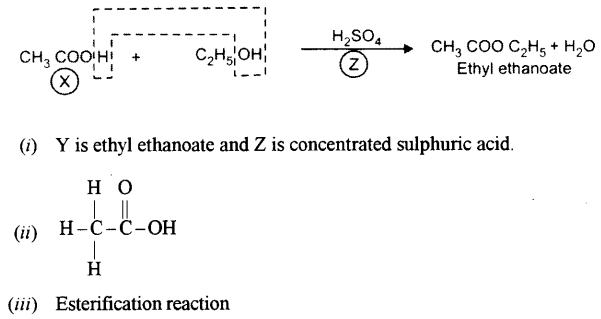(b) Ethane bums in oxygen to form CO2 and H2O according to the equation .
2C2H6 + 7O→ 4CO2 + 6H2O
If 1250 cc of oxygen is burnt with 300 cc of ethane.
Calculate:
(i) the volume of CO2 formed.
(ii) the volume of unused CO2.
(i)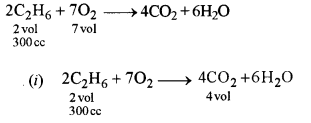2 vol. of ethane forms 4 volumes of carbon dioxide 4
1 vol. of ethane forms $$\frac{4}{2}$$ volumes of carbon dioxide.

(ii) 2 vol. of ethane requires 7 volumes of oxygen
2 vol. of ethane requires $$\frac{7}{2}$$ volumes of oxygen
300 cc of ethane requires $$\frac{7}{2} \times 300=1050 \mathrm{cc}$$
Total volume of Oxygen = 1250 cc
Volume of oxygen used = 1050 cc
Unused oxygen =(1250-1050) cc
= 200cc

(c) Three solutions P, Q and R have pH value of 3.5, 5.2 and 12.2 respectively. Which one of these is a :
(i) Weak acid?
(ii) Strong alkali ?
(c) P=3.5
Q=5.2
R= 12.2(i) Weak acid = Q
(ii) Strong alkah = R

Question 7.
(a) Give a chemical test to distinguish between the following pairs of chemicals: 
(i) Lead nitrate solution and Zinc nitrate solution.
(ii) Sodium chloride solution and Sodium nitrate solution.
(i) Add ammonium hydroxide to the solutions of lead nitrate and zinc nitrate dropwise and then in excess.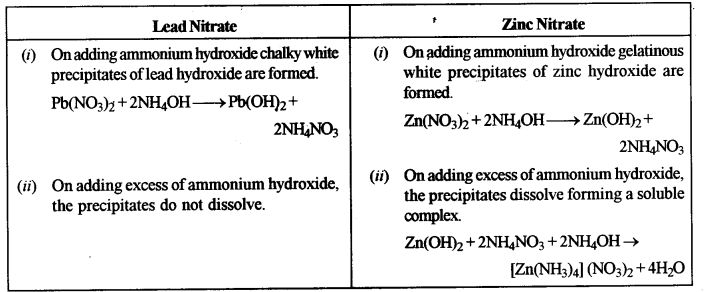(ii) Add silver nitrate solution to both sodium chloride and sodium nitrate solution and observe.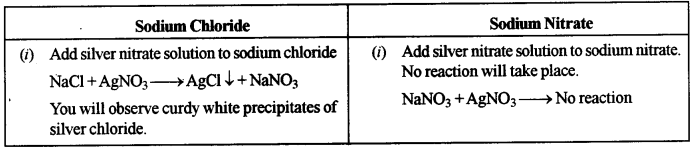(b) Write a balanced equation for the preparation of each of the following salts : 
(i) Copper sulphate from Copper carbonate
(ii) Zinc carbonate from Zinc sulphate.
(i) CUCO3 + H2SO4 → CuSO4 + H2O + CO2
(ii) ZnSO4 + Na2CO3 → ZnCO3 + Na2SO4

(c) (i) What is the type of salt formed when the reactants are heated at a suitable temperature for the preparation of Nitric acid ? 
(ii) State why for the preparation of Nitric acid, the complete apparatus is made up of glass.